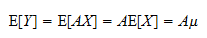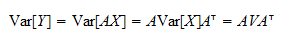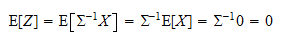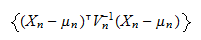StatLect

Slutsky's theorem

Slutsky's theorem concerns the convergence in distribution of the transformation of two sequences of random vectors, one converging in distribution and the other converging in probability to a constant.Joint convergence in distribution

Slutsky's theorem is based on the fact that if a sequence of random vectors converges in distribution and another sequence converges in probability to a constant, then they are jointly convergent in distribution.

Proposition (Joint convergence) Letandbe two sequences of random vectors. Ifand, whereis a constant, thenProof

In the proposition above we have indicated convergence in probability byand convergence in distribution by.

The theorem

We provide a statement of Slutsky's theorem that is slightly more general than the statement usually found in standard references.

Proposition (Slutsky) Letandbe two sequences of random vectors such thatand, whereis a constant. Letbe a continuous function. Then,Proof

The coupleis jointly convergent in distribution toby the proposition above (Joint convergence). Therefore, by the Continuous Mapping theorem, the fact thatis continuous implies thatconverges in distribution to.

The theorem is valid also whenandare sequences of random matrices (the reason being that random matrices can be thought of as random vectors whose entries have been re-arranged into several columns).

Implications

Since the sum and the product are continuous functions of their operands, Slutsky's theorem implies thatwhen,and the dimensions ofandare such that their sum and/or their product are well-defined.

Solved exercises

Below you can find some exercises with explained solutions.

Exercise 1

Letbe a sequence ofrandom vectors such thatwhereis a normal random vector with meanand invertible covariance matrix.

Letbe a sequence ofrandom matrices such thatwhereis a constant matrix. Find the limit in distribution of the sequence of products.

Solution

By Slutsky's theoremwhereThe random vectorhas a multivariate normal distribution, because it is a linear transformation of a multivariate normal random vector (see the lecture entitled Linear combinations of normal random variables). The expected value ofisand its covariance matrix isTherefore, the sequence of productsconverges in distribution to a multivariate normal random vector with meanand covariance matrix.

Exercise 2

Letbe a sequence ofrandom vectors such thatwhereis a normal random vector with meanand invertible covariance matrix.

Letbe a sequence ofrandom matrices such thatFind the limit in distribution of the sequenceSolution

By the Continuous Mapping theoremTherefore, by Slutsky's theoremUsing the Continuous mapping theorem again, we getSinceis an invertible covariance matrix, there exists an invertible matrixsuch thatTherefore,where we have definedThe random vectorhas a multivariate normal distribution, because it is a linear transformation of a multivariate normal random vector (see the lecture entitled Linear combinations of normal random variables). The expected value ofisand its covariance matrix isThus,has a standard multivariate normal distribution (meanand variance) andis a quadratic form in a standard normal random vector. So,has a Chi-square distribution withdegrees of freedom. In summary, the sequenceconverges in distribution to a Chi-square distribution withdegrees of freedom.

Exercise 3

Let everything be as in the previous exercise, except for the fact that nowhas mean. Find the limit in distribution of the sequencewhereis a sequence ofrandom vectors converging in probability to.

Solution

DefineBy Slutsky's theoremwhereis a multivariate normal random variable with meanand variance. Thus, we can use the results of the previous exercise on the sequencewhich is the same asand we find that it converges in distribution to a Chi-square distribution withdegrees of freedom.

References

van der Vaart, A. W. (2000) Asymptotic Statistics, Cambridge University Press.

The book

Most of the learning materials found on this website are now available in a traditional textbook format.

Glossary entries
Share